ANALYTIC APPROXIMATION FOR THE PROBABILITY

THAT A PORTFOLIO SURVIVES FOREVER

Bernard J. McCabe

Daniel H. Wagner Associates, Malvern PA

We give an approximate formula for the probability that a portfolio with a certain random return and fixed spending survives forever. This is useful as a conservative way of being sure that a portfolio will outlive an individual, no matter how long the lifetime. It could also be used in planning by a charitable trust to ensure that it safely survives.

Key Words: Financial planning, portfolio analysis, Monte Carlo, lognormal distribution.

Introduction

Consider a portfolio which is invested in a way so that the expected return m stays constant over the years, and the spending or disbursements is also constant, corrected for inflation. If the return was not subject to variability or uncertainty then the investor could assure himself that the portfolio would last forever by simply spending less than the return (net of inflation). But realistically the return is uncertain. It may hover around m, and average out to be m over the years, but it varies up and down around m, and the size of the differences is measured by the standard deviation s. There are a number of ways to think about s:

• s is the size of a typical discrepancy
• the return has about an equal chance to be in or out of the interval (m – 1.67s, m + 1.67s)
• there is probability .68 that the return is in the interval ms and m + s.
So given that the portfolio is characterized by the mean m and the standard deviation s, the issue we examine here is, what is the chance that the portfolio will last indefinitely, with annual disbursements s? Clearly s must be less than m, if there is non-zero uncertainty in the return. Intuitively we reason that the amount less must depend on the magnitude of s. But what specifically is the relationship, and what is its stability? That is, does the safe level of spending depend sharply on s, or is it a gentle slowly varying relationship.

It is also the case that we would like to derive simple formulas for the key event, the probability that the portfolio survives, formulas that can be graphed or used in spreadsheet calculations. Well, wanting to have such formulas and having them are often two different things! But in this case we are fortunate: the formulas can be derived. They are not terribly simple, but they are explicit, and can be programmed on a spreadsheet.

Finally we mention that for detailed planning one may need to resort to a Monte Carlo spending planner such as the one discussed in McCabe (1999). The results here are intended to be insightful, and useful, but they cannot substitute for a detailed examination of an individual’s situation when that involves time varying effects due to variable income and tax status.

The main result

Figure 1 shows our main result, for some typical values of mean return and standard deviation, actually the same values that were analyzed in McCabe (1999). In the figure we show the probability that the portfolio will survive forever as a function of the percentage spending s. That is, s is expressed as a percentage of the initial portfolio value. Note that the survival probability dependence on s is rather gentle, although it does become sharper with the more conservative investments.

There are a couple of interesting things to be learned from an examination of this plot.

• No matter how small spending is, there is always some chance the portfolio will be wiped out – a couple of bad years followed by no reduction in spending will do it.
• The other side of that coin: an unreasonably high spender may survive with some good luck.
• If spending is exactly the same as return, as a percentage, then there is about a 50% chance the portfolio will survive forever.
• To have some high chance of surviving forever, say 90%, then spending must be less than return m, and less by an amount that grows with s. The precise amount can be determined using the formulas below.
Here are the general formulas: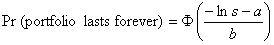where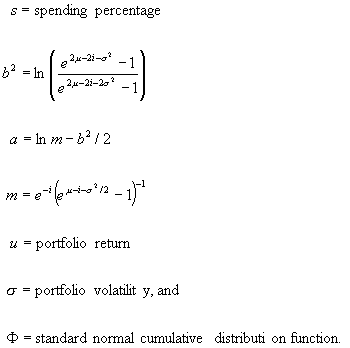Applicability and Future Research Directions

These results raise some interesting questions for further research.

1. What happens if spending can be modified each and every year (easier for the charitable fund perhaps than for an individual) in reaction to changing fortunes? Then the question is not whether the fund will last forever (it will, with probability 1) but what will average spending be. This in turn raises an interesting issue with respect to disbursement from trusts. Recently, UniTrusts have become much more fashionable, in part because they key their distribution to some fraction of the corpus of the trust rather than limit them to accounting income. A revised version of the formulation discussed above might be used to determine the likelihood that there will remain a sufficient amount of principal for remainderment when the flows to income beneficiaries stops.
2. As a even more specific example, one might consider using this analysis to look into charitable remainder trusts. In those instances, an individual or group of individuals gives securities to a trust designed to provide them with some ongoing income flow for a term fixed or determined by their death and to leave the principal in the trust at that time to charity. As they constitute a useful tax-planning tool, charitable remainder trusts have become popular as a means of sheltering capital gains in greatly appreciated securities. The analysis proposed here might be used to evaluate more precisely what the actual chances are that the principal in the trust upon the termination of the income to beneficiaries is sufficient in proportion of the original gift to satisfy the original charitable intents. The analysis is made more complex by the need to provide for both uncertainty of return and uncertainty of maturity (although standard mortality tables might be used for the sake of simplicity).
3. A further element of complexity in the analysis arises if uncertainty in return is itself uncertain. Capital market history does clearly suggest that the volatility of returns is not constant over time, both in absolute terms and relative to the returns actually achieved. One might consider modeling it (or preferably its logarithm) as a stationary stochastic process and analyzing the resulting stochastic equation. Traditionally, risk has been measured in terms of the volatility of returns, but that approach does not meet the needs of most individual investors. Downside risk protection has become a much more important element of the appreciation of risk, as it is the one to which individuals have been repeatedly proven to respond most (see Bernadzi and Thaler (1995) or Brunel (1998)). The ultimate expression of that downside risk is found when the principal in the portfolio is exhausted. At that time, very much as the gambler who has run out of money to keep on gambling, the investor is left with no opportunity to participate in any future upside potential. The analysis discussed here is an initial stab at getting at that issue.
Appendix on the mathematical derivation

Suppose we start with a portfolio of size A0. We assume each year i that the portfolio return (as a multiplier) is an independent draw from a lognormal distribution, Li = exp(Xi), where Xi is normal with mean m and standard deviation s, for i = 1, 2, … Suppose each year that an amount s is withdrawn from the portfolio. So the portfolio size in year n + 1 satisfies the stochastic update equation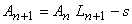It is convenient to assume that A0 = 1 and that s is expressed as a percent of A0. The return m is assumed to be net of inflation. Thus the spending level s can remain constant since it is expressed in constant year 0 currency. Note in the definition of An we do not make 0 an absorbing state – it is unnecessary if we make the convention that An < 0 is equivalent to the portfolio not surviving. This is permissible because once An is negative so are all subsequent values: by definition An+k < 0 if An < 0, for all k, since Ln > 0. Note also that s should represent total spending including whatever will be needed to pay taxes each year.

Under the above assumptions we are interested in calculating the probability a that the portfolio survives forever,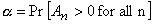Expanding the expression for An we have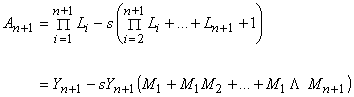where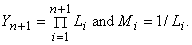Note that Mi is also lognormal, but with parameters –m and s. For facts about the lognormal distribution, in particular the formulas for its moments that we will be using, see Johnson and Kotz (1970), Chapter 14.

So,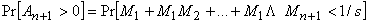and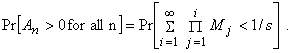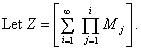Since Z = M1(1 + M2 + M2M3 + … ) we see that Z has the same distribution as a random variable of the form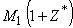where M1 and Z* are independent of each other, and Z* has the same distribution as Z.

We can use that equality-in-distribution relationship to set up equations for the first two moments of Z. Those will be used in turn to approximateLet m denote the mean of Z and t its standard deviation. Then,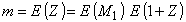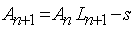and we can solve for m to obtainAlso, b = E (Z2) must satisfy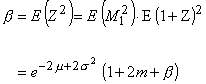and hence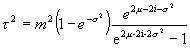Monte Carlo experiments simulating the random variable Z show that its distribution is close to being lognormal. Note that Z is defined by an infinite sum. We first determined that the distribution of Z does not change appreciably past 50 terms of that infinite sum; so, to be on the safe side, for the results presented we take 100 terms of that infinite sum. Figures 2 and 3 show a typical density and cumulative, corresponding to
one of the standard portfolios used in McCabe (1999), and 10,000 replications.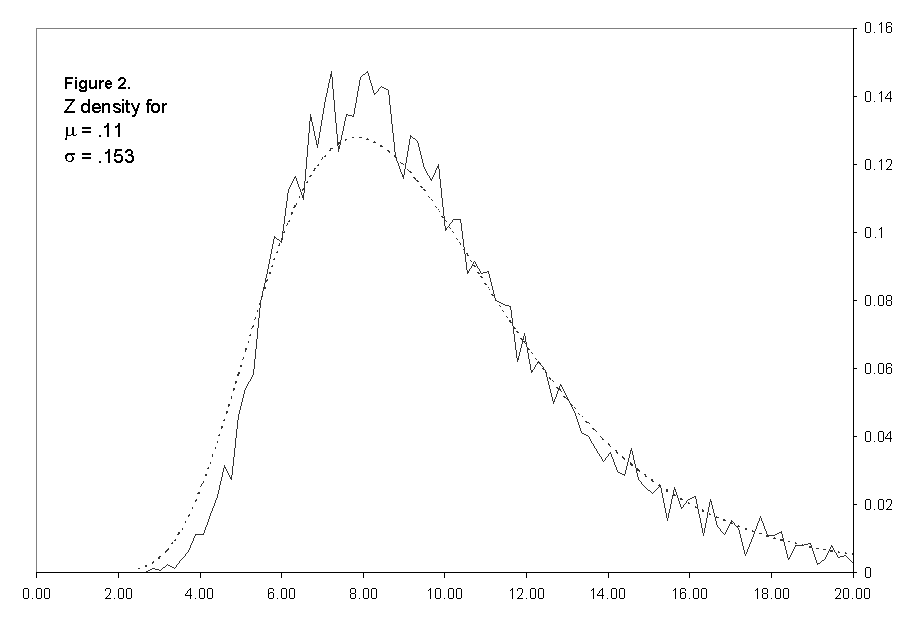Figure 2: Comparison of Monte Carlo to Lognormal Fit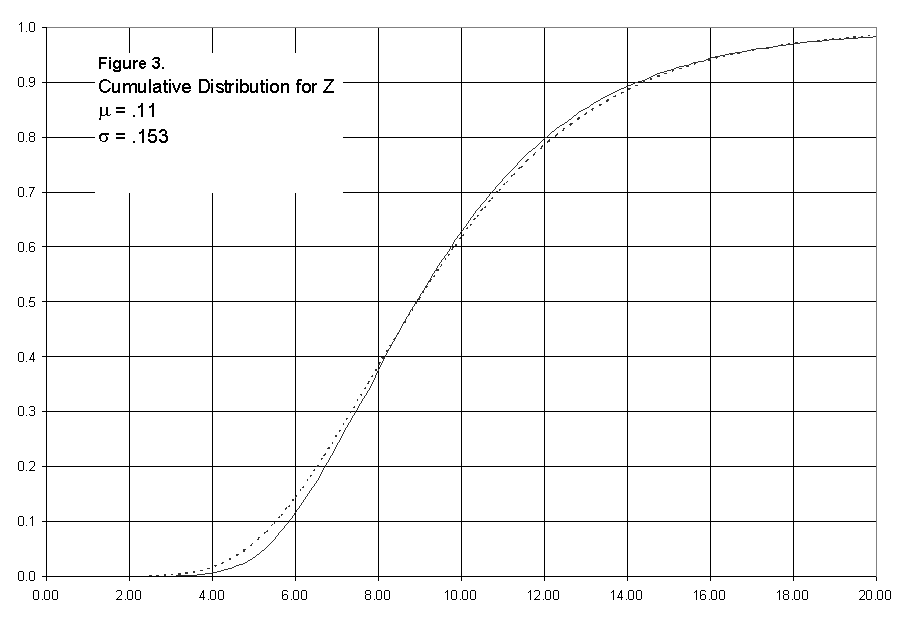Figure 3: Cumulative Distribution for Z

So, for numerical purposes we are justified in assuming that Z is exactly

lognormal, of the form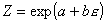where e is a standard (0,1) normal random variable and some unknown constants a and b. Knowing the mean and variance of Z, we can solve for a and b. To do that we use the relationships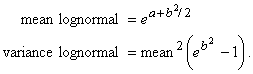So we have,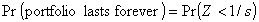wherePlots of this for various values of s and our standard five portfolios are shown in Figure 1.

REFERENCES

Brunel, J. (1998): A Second Look at Absolute Returns, Journal Of Private Portfolio Management, Vol. 1, No. 1.

Benartzi, S. and Thaler, R. H. (1995): Myopic Loss Aversion and the Equity Premium Puzzle, Quarterly Journal of Economics, pp. 73-92.

McCabe, B. J. (1999): Monte Carlo Analysis of the Impact of Portfolio Volatility on After-Tax Wealth, to appear in Journal of Private Portfolio Management.

Johnson, N. L. and Kotz, S. (1970): Continuous Univariate Distributions – 1, Houghton Mifflin.

Bernard McCabe is a Senior Vice President with the consulting firm Daniel H. Wagner Associates in Malvern PA. He was part of the team who developed R\$P, a retirement planning program which considers uncertainty in portfolio returns.